Mrli

Contacts:
QQ博客园

# 李宏毅机器学习_摘要

2020/12/20 机器学习
Word count: 15,560 | Reading time: 64min

## P3Regression - Case Study

### regularization解决overfitting(L2正则化解决过拟合问题)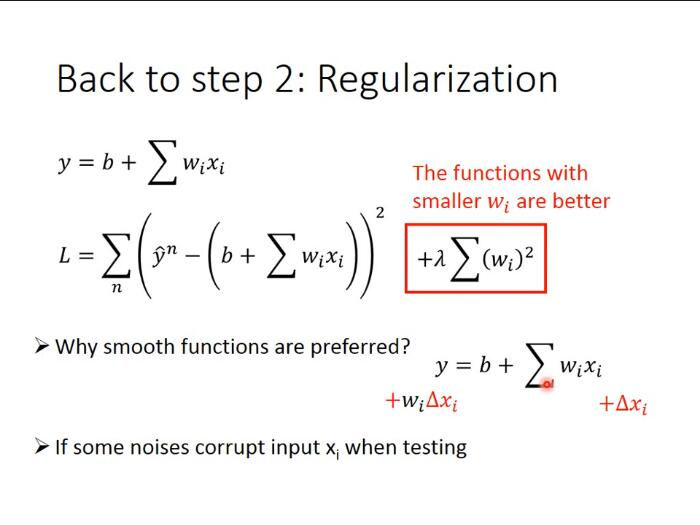regularization可以使曲线变得更加smooth，training data上的error变大，但是 testing data上的error变小。有关regularization的具体原理说明详见下一部分

λ值越大代表考虑smooth的那个regularization那一项的影响力越大，我们找到的function就越平滑

## P4Basic Concept

### bias和variance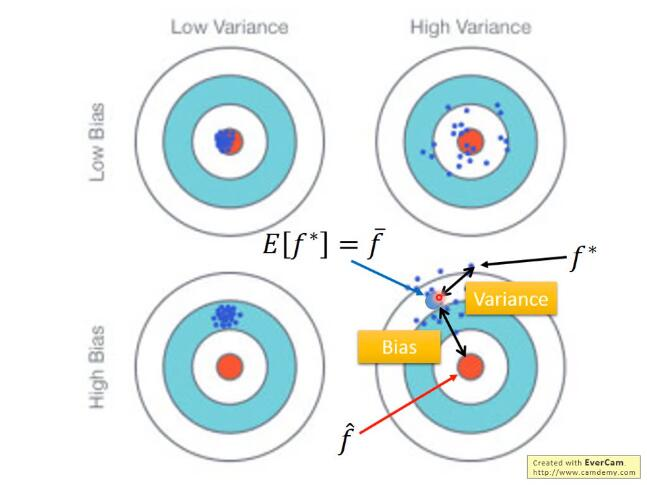bias表示离靶心的距离, 而variance表示散布情况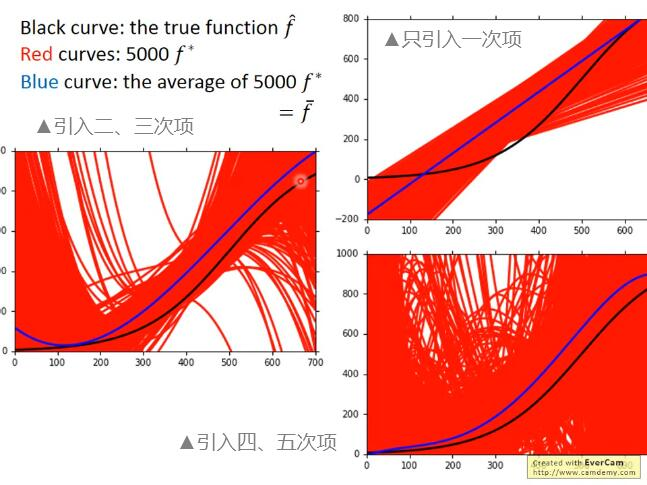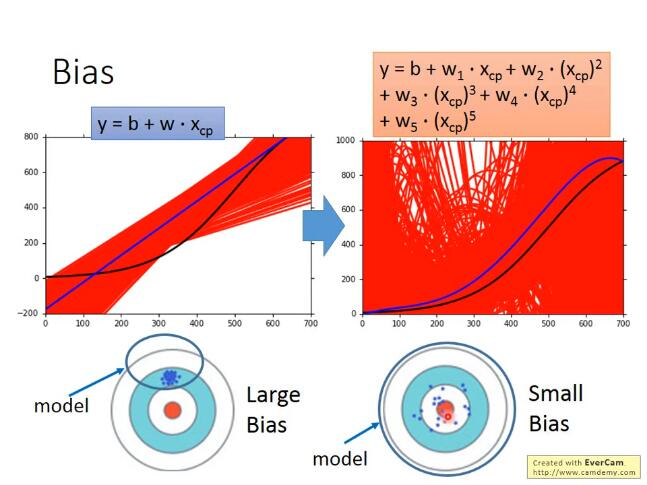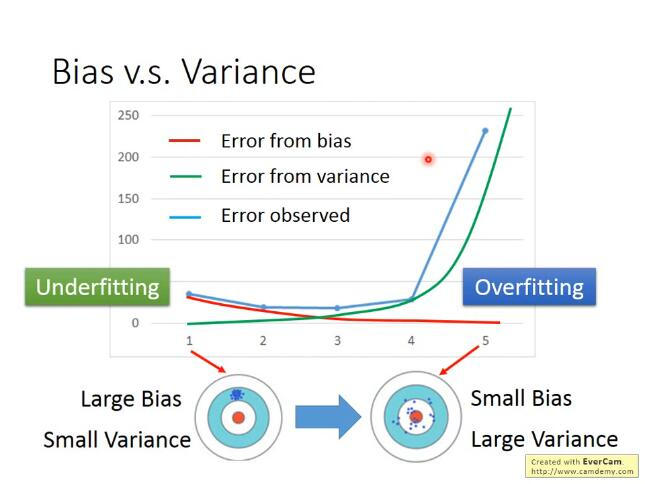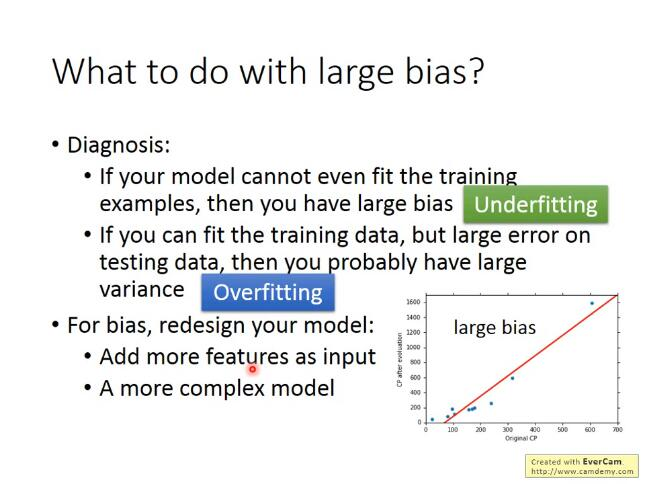bias大： 如果无法fit训练数据, 证明是欠拟合;如果能fit训练数据，但是在测试数据上有较大的误差，那么就是过拟合——做法：增大特征or增大次项

variance大： 更多的数据（数据增强）or 正则化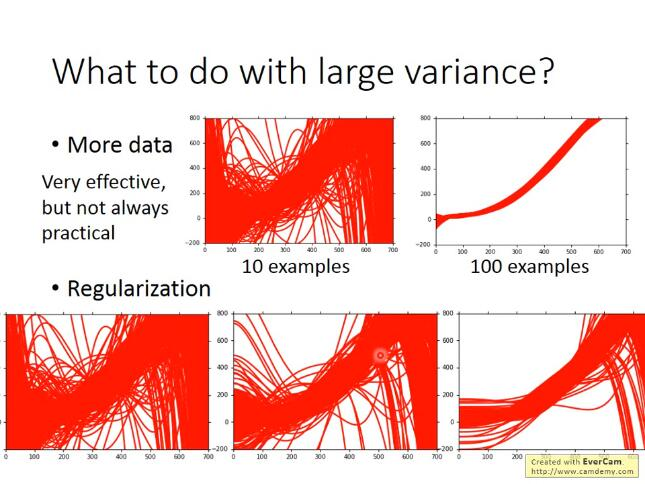### 交叉验证：#### N-fold cross validation （十折交叉验证）$\theta^{*}=\arg \min _{\theta} L(\theta)$

L: loss function
$\theta$ : parameters(上标表示第几组参数, 下标表示这组参数中的第几个参数)##### 最基本、最简单的大原则是：learning rate通常是随着参数的update越来越小的

Divide the learning rate of each parameter by the root mean square(方均根) of its previous derivatives

### Feature Scaling

##### 概念介绍##### scaling method:

z-score:## 6. P10Classification_1

Regression的output是连续性质的数值，而classification要求的output是离散性质的点，我们很难找到一个Regression的function使大部分样本点的output都集中在某几个离散的点附近

#### Solution：Generative model——朴素贝叶斯分类器

![function](李宏毅机器学习-摘要/P6 classification\function.jpg)

![beyas](李宏毅机器学习-摘要/P6 classification\beyas.jpg)

#### 高斯分布

$f_{\mu, \Sigma}(x)=\frac{1}{(2 \pi)^{D / 2}} \frac{1}{|\Sigma|^{1 / 2}} \exp \left\{-\frac{1}{2}(x-\mu)^{T} \Sigma^{-1}(x-\mu)\right\}$

#### 最大似然估计_参数估计

![最大似然估计](李宏毅机器学习-摘要/P6 classification\最大似然估计.jpg)

![估计出参数](李宏毅机器学习-摘要/P6 classification\估计出参数.jpg)

![预测](李宏毅机器学习-摘要/P6 classification\预测.jpg)

===> 训练结束：再把testing data上得到的结果可视化出来，即右下角的图，发现分的不是太好，正确率才是47%我们之前用的只是Defense和SP Defense这两个参数，在二维空间上得到的效果不太好，但实际上一开始就提到了宝可梦总共是有6个features的，也许在二维空间上它们是重叠在一起的，但是在六维空间上看它们也许会分得很好，每一个宝可梦都是六维空间中的一个点，于是我们的是一个6-dim的vector，则是一个6*6的matrix，发现得到的准确率也才64%，这个分类器表现得很糟糕，是否有办法将它改进的更好？

### 案例总结

machine learning可以概况为以下三个step

![threeSteps](李宏毅机器学习-摘要/P6 classification\threeSteps.jpg)

![threeSteps笔记](李宏毅机器学习-摘要/P6 classification\threeSteps笔记.jpg)

![概率分布](李宏毅机器学习-摘要/P6 classification\概率分布.jpg)

#### Analysis Posterior Probability分析后置概率的表达式$P\left(C_{1} \mid x\right)=\sigma(w \cdot x+b)$这个式子就解释了，当class 1和class 2共用的时候，它们之间的boundary会是linear的

## 7. P11Logistic Regression

### Three Steps of machine learning

#### Step 1：function set#### Step 2：Goodness of a function$\begin{array}{l} \qquad w^{*}, b^{*}=\arg \max _{w, b} L(w, b)=\arg \min _{w, b}(-\ln L(w, b)) \\ -\ln L(w, b)=-\ln f_{w, b}\left(x^{1}\right) \\ \quad-\ln f_{w, b}\left(x^{2}\right) \\ \quad-\ln \left(1-f_{w, b}\left(x^{3}\right)\right) \\ \quad-\ldots \end{array}$

\begin{aligned} -\ln L(w, b)=&-\left[\hat{y}^{1} \ln f_{w, b}\left(x^{1}\right)+\left(1-\hat{y}^{1}\right) \ln \left(1-f_{w, b}\left(x^{1}\right)\right)\right] \\ &-\left[\hat{y}^{2} \ln f_{w, b}\left(x^{2}\right)+\left(1-\hat{y}^{2}\right) \ln \left(1-f_{w, b}\left(x^{2}\right)\right)\right] \\ &-\left[\hat{y}^{3} \ln f_{w, b}\left(x^{3}\right)+\left(1-\hat{y}^{3}\right) \ln \left(1-f_{w, b}\left(x^{3}\right)\right)\right] \\ &-\ldots \end{aligned}

$-\ln L(w, b)=\sum_{n}-\left[\hat{y}^{n} \ln f_{w, b}\left(x^{n}\right)+\left(1-\hat{y}^{n}\right) \ln \left(1-f_{w, b}\left(x^{n}\right)\right)\right]$

##### 交叉熵cross entropy交叉嫡的含义是表达这两个distribution有多接近, 如果p和q这两个distribution一模一样的话, 那 它们算出来的cross entropy就是0(详细解释在“信息论"中), 而这里 $f\left(x^{n}\right)$ 表示function的output, $\hat{y}^{n}$ 表示预期 的 target, 因此交叉嫡实际上表达的是希望这个function的output和它的target越接近越好

$w^{*}, b^{*}=\arg \max _{w, b} L(w, b)=\arg \min _{w, b}\left(-\ln L(w, b)=\sum_{n}-\left[\hat{y}^{n} \ln f_{w, b}\left(x^{n}\right)+\left(1-\hat{y}^{n}\right) \ln \left(1-f_{w, b}\left(x^{n}\right)\right)\right]\right.$

#### step 3：Find the best function$\theta^{*}=\arg \min _{\theta} L(\theta)$

L: loss function
$\theta$ : parameters(上标表示第几组参数, 下标表示这组参数中的第几个参数)

$w_{i}=w_{i}-\eta (\sum_{n}-\left(\hat{y}^{n}-f_{w, b}\left(x^{n}\right)\right) x_{i}^{n})$

• learning rate, 是你自己设定的
• $\cdot x_{i},$ 来自于data
• $\hat{y}^{n}-f_{w, b}\left(x^{n}\right),$ 代表function的output跟理想target的差距有多大, 如果离目标越远, update的步伐就要越 大

### Logistic Regression V.s. Linear Regression##### compare in step1
• Logistic Regression是把每一个feature $x_{i}$ 加权求和, 加上bias, 再通过sigmoid function, 当做function的 output
• 因为Logistic Regression的output是通过sigmoid function产生的, 因此一定是介于0 1之间; 而linear Regression的output并没有通过sigmoid function, 所以它可以是任何值

### Logistic Regression + Square error？

Square error求微分情况:### Discriminative v.s. Generative

#### same model but different currency

Logistic Regression的方法，我们把它称之为discriminative的方法；而我们用Gaussian来描述posterior Probability这件事，我们称之为Generative的方法,

Generative model做的事情就是脑补，它会自己去想象一些事情，于是会做出一个和我们人类直觉想法不太一样的判断结果，就像toy example里，我们做了naive bayes这样一个假设(事实上我们并不知道这两个feature是否相互独立)，于是Naive bayes会在class 2里并没有出现过两个feature都是1的样本点的前提下，自己去脑补有这样的点

### Conclusion

\begin{aligned} P\left(C_{i} \mid x\right)=& \frac{P\left(C_{i}\right) P\left(x \mid C_{i}\right)}{\sum_{j=1}^{n} P\left(C_{j}\right) P\left(x \mid C_{j}\right)}(1) \\ &=\sigma(z)=\frac{1}{1+e^{-z}}=\frac{1}{1+e^{-\left(b+\sum_{k} w_{k} x_{k}\right)}} \end{aligned}

Generative model的好处是，它对data的依赖并没有像discriminative model那么严重，在data数量少或者data本身就存在noise的情况下受到的影响会更小，而它还可以做到Prior部分与class-dependent部分分开处理，如果可以借助其他方式提高Prior model的准确率，对整一个model是有所帮助的(比如前面提到的语音辨识)

### Multi-class Classification

#### softmax

softmax的意思是对最大值做强化，因为在做第一步的时候，对取exponential会使大的值和小的值之间的差距被拉得更开，也就是强化大的值

##### Limitation of Logistic Regression

Logistic Regression其实有很强的限制，给出下图的例子中的Training data，想要用Logistic Regression对它进行分类，其实是做不到的, 如异或

##### Feature Transformation## 8. P12 Brief Introduction of Deep Learning

### Ups and downs of Deep Learning

• 1958：Perceptron(linear model)，感知机的提出
• 和Logistic Regression类似，只是少了sigmoid的部分
• 1969：Perceptron has limitation，from MIT
• 1980s：Multi-layer Perceptron，多层感知机
• 和今天的DNN很像
• 1986：Backpropagation，反向传播
• Hinton propose的Backpropagation
• 存在problem：通常超过3个layer的neural network，就train不出好的结果
• 、1989: 1 hidden layer is “good enough”，why deep？
• 有人提出一个理论：只要neural network有一个hidden layer，它就可以model出任何的function，所以根本没有必要叠加很多个hidden layer，所以Multi-layer Perceptron的方法又坏掉了，这段时间Multi-layer Perceptron这个东西是受到抵制的
• 2006：RBM initialization(breakthrough)：Restricted Boltzmann Machine，受限玻尔兹曼机
• Deep learning -> another Multi-layer Perceptron ？在当时看来，它们的不同之处在于在做gradient descent的时候选取初始值的方法如果是用RBM，那就是Deep learning；如果没有用RBM，就是传统的Multi-layer Perceptron
• 那实际上呢，RBM用的不是neural network base的方法，而是graphical model，后来大家试验得多了发现RBM并没有什么太大的帮助，因此现在基本上没有人使用RBM做initialization了
• RBM最大的贡献是，它让大家重新对Deep learning这个model有了兴趣(石头汤的故事)
• 2009：GPU加速的发现
• 2011：start to be popular in speech recognition，语音识别领域
• 2012：win ILSVRC image competition，Deep learning开始在图像领域流行开来### Neural Network

#### concept### Network Structure

• input的地方，叫做input layer，输入层(严格来说input layer其实不是一个layer，它跟其他layer不一样，不是由neuron所组成的)
• output的地方，叫做output layer，输出层
• 其余的地方，叫做hidden layer，隐藏层
• 激活函数: 每一个neuron里面的sigmoid function，在Deep Learning中被称为activation function(激励函数)，事实上它不见得一定是sigmoid function，还可以是其他function(sigmoid function是从Logistic Regression迁移过来的，现在已经较少在Deep learning里使用了)

★.network structure+neuron该如何连接在一起的含义: 其实是define了一个function set(model)

### Feedforward network▲.全连接: 因为layer和layer之间，所有的neuron都是两两连接，所以它叫Fully connected的network；因为现在传递的方向是从layer 1->2->3，由后往前传，所以它叫做Feedforward network#### Output Layer

output layer做的事情，其实就是把它当做一个Multi-class classifier，它是拿经过feature extractor转换后的那一组比较好的feature(能够被很好地separate)进行分类的，由于我们把output layer看做是一个Multi-class classifier，所以我们会在最后一个layer加上softmax

### 整个手写体识别分析:

input固定为256维(图片)，output固定为10维的feedforward neural network，实际上这个network structure就已经确定了一个function set(model)的形状，在这个function set里的每一个function都可以拿来做手写数字识别，接下来我们要做的事情是用gradient descent去计算出一组参数，挑一个最适合拿来做手写数字识别的function

#### Step 1：Neural Networkintput输入为16*16的图片(256维) -->经过神经网络(函数) --> 输出10维的vector --> 经过softmax函数选出可能性最大的结果输出-output

#### Step 2：Goodness of functioninput这张image的256个pixel，通过这个neural network之后，会得到一个output，称之为y；而从这张image的label中转化而来的target，称之为，有了output 和target 之后，要做的事情是计算它们之间的cross entropy(交叉熵)，这个做法跟我们之前做Multi-class classification的时候是一模一样的—> 分类问题多使用交叉熵

Cross Entropy $: l(y, \hat{y})=-\sum_{i=1}^{10} \hat{y}_{i}$lny$_{i}$

### Design network structure V.s. Feature Engineering(网络结构设计 vs 特征工程)

deep learning: 通过network 找到一组合适的param来训练, 让机器自动学会如何提取特征==>获得model

### P15Why Deep-Q:参数变多,是变宽还是变深呢? 是不是暴力的方法呢?

A:

• 论文比较相同参数, 深度效果好于宽度;
• DNN结构一个很大的优势是，Modularization(模块化)，它用的是结构化的架构

#### Modularization

• 理解效果：模组化： 如分类长发的女孩、长头发的男孩、短发的女孩、短发男孩。

​ 如果按照shallow network的想法，我们分别独立地train四个classifier(其实就相当于训练四个独立的model)，然后就可以解决这个分类的问题。但是显而易见的是长头发的男生样本会非常少，没有太多的training data，所以，你train出来的classifier就比较weak，去detect长头发男生的performance就比较差

![模组化](李宏毅机器学习-摘要/P12\模组化.jpg• Classifier1：检测是男生或女生
• Classifier2：检测是长头发或短头发#### deep -> modularization

• 第一层neuron，它是一个最basic的classifier，检测的是颜色、线条这样的小特征
• 第二层neuron是比较复杂的classifier，它用第一层basic的classifier的output当作input，也就是把第一层的classifier当作module，利用第一层得到的小特征分类出不同样式的花纹
• 而第三层的neuron又把第二层的neuron当作它module，利用第二层得到的特征分类出蜂窝、轮胎、人
• 以此类推#### Conclusion

Deep总结：

• 考虑input之间的内在关联，所有的class用同一个model来做分类
• modularization思想，复杂问题简单化，把检测复杂特征的大任务分割成检测简单特征的小任务
• 所有的classifier使用同一组参数的子特征检测器，共享检测到的子特征
• 不同的classifier会share部分的参数和data，效率高
• 联系logic circuit和剪纸画的例子
• 多层hidden layer对complex问题的处理上比较有优势

## 9. P13Backpropagation

Backpropagation是神经网络中用来做gradient descent的方法， 目的是使优化函数尽可能得变成我们想要的， 假设我们现在要处理的是分类任务，loss function为交叉熵， total loss为$L(\theta)=\sum_{n=1}^{N} l^{n}(\theta)$

$\frac{\partial L(\theta)}{\partial w}=\sum_{n=1}^{N} \frac{\partial l^{n}(\theta)}{\partial w}$### Forward pass

• 比如input layer作为neuron的输入时，前面连接的是，所以微分值就是；前面连接的是，所以微分值就是
• 比如hidden layer作为neuron的输入时，那该neuron的input就是前一层neuron的output，于是的值就是前一层的z经过activation function之后输出的值(下图中的数据是假定activation function为sigmoid function得到的)### Backward pass

$\frac{\partial l}{\partial z}=\frac{\partial a}{\partial z} \frac{\partial l}{\partial a}$

$\frac{\partial l}{\partial a}=\frac{\partial z^{\prime}}{\partial a} \frac{\partial l}{\partial z^{\prime}}+\frac{\partial z^{\prime \prime}}{\partial a} \frac{\partial l}{\partial z^{\prime \prime}}$

$\frac{\partial l}{\partial z}=\frac{\partial a}{\partial z} \frac{\partial l}{\partial a}=\sigma^{\prime}(z)\left[w_{3} \frac{\partial l}{\partial z^{\prime}}+w_{4} \frac{\partial l}{\partial z^{\prime \prime}}\right]$case 1: Output Layer

$\frac{\partial l}{\partial z^{\prime}}=\frac{\partial y_{1}}{\partial z^{\prime}} \frac{\partial l}{\partial y_{1}}$

$\frac{\partial l}{\partial y_{1}}$ 就是loss function对 $y_{1}$ 的偏微分, 它取决于你的loss function是怎么定义的, 也就是你的output和target之间是怎么 evaluate的, 你可以用cross entropy, 也可以用mean square error, 用不同的定义, $\frac{\partial l}{\partial y_{1}}$ 的值就不一样

function的微分 $\sigma^{\prime}(z),$ 就可以得到output $\frac{\partial l}{\partial z}$▲注: 如果是正向做Backward pass的话, 实际上每次计算一个 $\frac{\partial l}{\partial z},$ 就需要把该neuron后面所有的 $\frac{\partial l}{\partial z}$ 都给计算一 遍, 会造成很多不必要的里复运算, 如果写成code的形式, 就相当于调用了很多次里复的函数; 而如果是反向做 Backward pass，实际上就是把这些调用函数的过程都变成调用“值"的过程, 因此可以直接计算出结果, 而不需要 占用过多的堆栈空间

### Summary

Forward pass，每个neuron的activation function的output，就是它所连接的weight的

Backward pass，建一个与原来方向相反的neural network，它的三角形neuron的output就是Author: Mrli